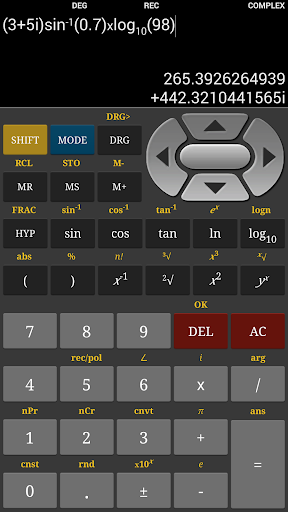# Scientific Calculator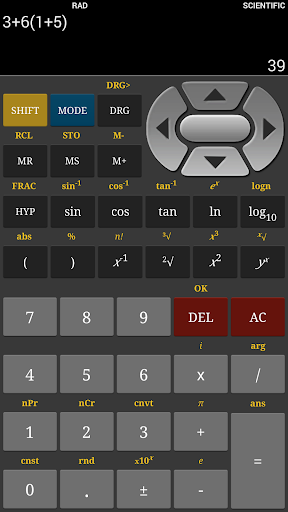Scientific Calculator - Scientific and Graphing Calculator

This is a fully featured scientific and graphing calculator which supports graph, matrix, complex numbers, equation solver and unit converter. For everyday calculations, the calculator also supports a basic mode.

Features:

* Large input/output display

* Dedicated Graph Plotter

* Complex number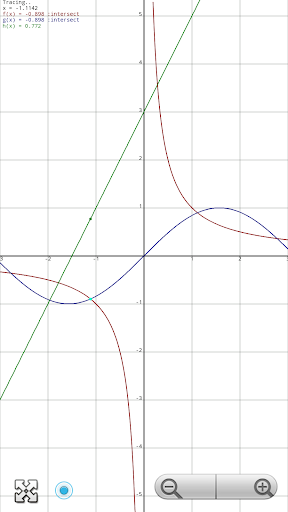* Full Matrix Support up to order of 9x9

* Simultaneous linear equation solver up to 9 variables

* Polynomial equation solver - gives both real and complex solution

* Landscape Mode

* Calculation History

* All Trigonometric operations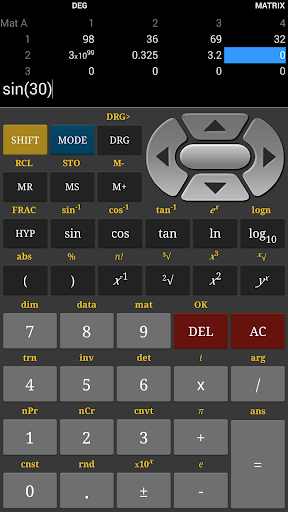* Binary, Decimal, Octal, Hexadecimal operation

* Bitwise operations

* Ten memory locations

* Results history feature

* Constants Table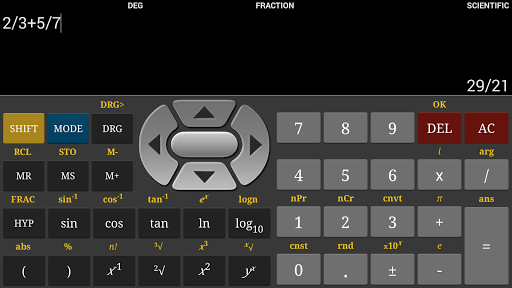* Built-in help (accessible via options menu)

You can also try the free version: